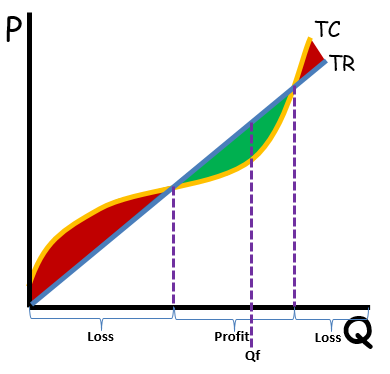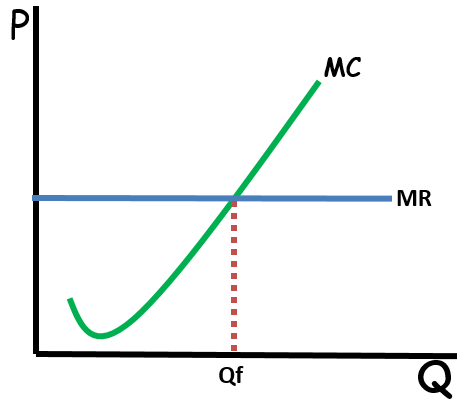### ReviewEcon.com

AP, IB, and College Microeconomic and Macroeconomic Principles

# Cost, Profit, and Revenue Run-Down

Updated 9/13/2021 Jacob Reed
Here is a run-down of the difference between accounting profit and economic profit. Since this is an economics website and you’re taking an economic principles class, economic profit is clearly the more important concept. But make sure you remember accounting profit on exam day too!After you read through this, practice your calculations with the 20 question Cost, Profit, and Revenue Review game.

Revenue
Revenue is money a firm brings in from sales. Total Revenue is Price times Quantity (TR = P x Q). You already learned about total revenue in the elasticity review

Marginal revenue is the change in Total Revenue divided by the change in Quantity (MR = ∆TR/∆Q). Most often the change in quantity is just one, so marginal revenue is usually the revenue a firm brings in for producing one more unit of output.

Costs
There are two types of costs you need to know in microeconomics. Explicit costs and implicit costs. These ideas were touched on in the opportunity cost review, but here we are going to use terminology as it relates to a firm (business).

Explicit cost: Explicit costs are the out of pocket costs paid by the business owner. Explicit costs for this website are the hosting fees, the cost of the software, etc.

Implicit cost: Implicit costs are the implied costs, or the value of opportunities lost (aside from out of pocket money costs). The implicit costs associated with producing this website include the value of my evening leisure time.

Profit
In microeconomics there are actually two types of profit you need to know: accounting profit and economic profit.

Accounting Profit: Accounting Profit is what most people think of as profit and although it isn’t nearly as important as economic profit, it shows up on many economics exams.

Accounting profit is total revenue minus explicit costs. For this website, accounting profit would be the revenue generated for the ads minus the web hosting fees and the cost of the software.

Note: Accounting profit is always higher than economic profit.

Economic Profit: Economic Profit is much more important to economists than accounting profit. Economic profit is Total Revenue minus Explicit and Implicit costs. So for this website, economic profit would subtract not only the hosting and software costs but also the cost of my time (labor) for creating the content.

On every one of the firm graphs, both implicit and explicit costs are contained within the cost curves. So if a firm is making a profit, it is an economic profit. If they are suffering a loss, it is an economic loss.  If they are making zero economic profit (breaking even), they are still making an accounting profit.

Breaking Even or Zero Economic Profit (AKA – Normal Profit)
When a firm is earning zero economic profit, its total revenue equals its total costs (both implicit and explicit). On the firm graphs, price will equal the average total cost (ATC). When that occurs, the entrepreneur will be earning whatever they could be earning doing the next best alternative. A teacher who quits teaching to become a street performer would break even (earn zero economic profit) if they earn a teacher’s salary by street performing. This person would still be earning an accounting profit. They would have to earn more than a teacher’s salary to be earning an economic profit.

Profit Maximization
One way to determine the profit maximizing quantity for a firm to produce is to look at total revenue versus total profit. Total revenue, for a perfectly competitive firm, will increase at a constant rate. The total cost will increase at varying rates. When total revenue is below total cost, the firm earns a negative economic profit. When total revenue equals total cost, the firm breaks even (earns zero economic profit). When the total revenue is greater than total cost, the firm earns a positive economic profit. Economic profit is maximized where total revenue is greater than total cost by the largest margin. In the graph, to the right, Qf is the profit maximizing quantity.Businesses follow this same logic. The only difference is that for a business the marginal benefit is called marginal revenue. A firm will maximize profit (total revenue – total cost) if they produce until marginal revenue (MR) is equal to marginal cost (MC). Producing less than the MR=MC quantity will mean MR is greater than MC so profit can be increased by producing more. Producing more than the MR=MC quantity means MC is greater than MR so profit will increase if less is produced. So, profit maximizing firms will continue to produce as long as the marginal revenue is greater than or equal to the marginal cost, but not produce any units where the marginal revenue is less than the marginal cost.Multiple Choice Connections:
2012 Released AP Microeconomics Exam Question: 28

Up Next:
Review Game: Cost, Revenue, and Profit quiz
Content Review Page: Cost Curves Review

Other recommended resources: Khan Academy (Economic vs accounting profit)